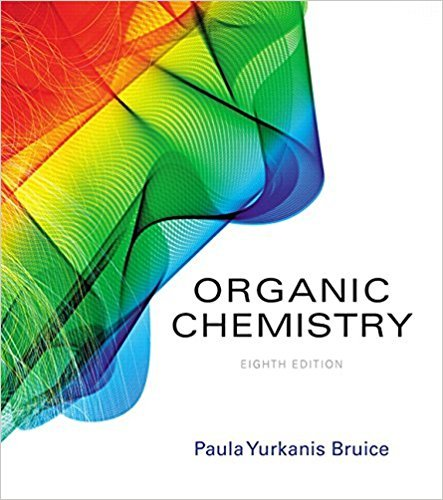×
Get Full Access to Organic Chemistry - 8 Edition - Chapter 5.6 - Problem 20
Get Full Access to Organic Chemistry - 8 Edition - Chapter 5.6 - Problem 20

×

# a. Calculate the percentage of isopropylcyclohexane molecules that have the isopropylISBN: 9780134042282 482

## Solution for problem 20 Chapter 5.6

Organic Chemistry | 8th Edition

• Textbook Solutions
• 2901 Step-by-step solutions solved by professors and subject experts
• Get 24/7 help from StudySoup virtual teaching assistantsOrganic Chemistry | 8th Edition

4 5 1 338 Reviews
23
2
Problem 20

a. Calculate the percentage of isopropylcyclohexane molecules that have the isopropyl substituent in anequatorial position at equilibrium. (Its G value at 25 C is -2.1 kcal/mol.)b. Why is the percentage of molecules with the substituent in an equatorial position greater forisopropylcyclohexane than for fluorocyclohexane?

Step-by-Step Solution:
Step 1 of 3

Devan Atteberry 4/14/2016 Chem 312 Week #3: Saturated/Unsaturated Hydrocarbons and Pi Bonds Major Structure at pH - Equilibrium A+ B= C+D o Equilibrium expression is Keq  Keq= (c) (d)/ (a) (b) or products/reactants...

Step 2 of 3

Step 3 of 3

##### ISBN: 9780134042282

Organic Chemistry was written by and is associated to the ISBN: 9780134042282. The answer to “a. Calculate the percentage of isopropylcyclohexane molecules that have the isopropyl substituent in anequatorial position at equilibrium. (Its G value at 25 C is -2.1 kcal/mol.)b. Why is the percentage of molecules with the substituent in an equatorial position greater forisopropylcyclohexane than for fluorocyclohexane?” is broken down into a number of easy to follow steps, and 44 words. Since the solution to 20 from 5.6 chapter was answered, more than 211 students have viewed the full step-by-step answer. This textbook survival guide was created for the textbook: Organic Chemistry, edition: 8. This full solution covers the following key subjects: . This expansive textbook survival guide covers 127 chapters, and 2116 solutions. The full step-by-step solution to problem: 20 from chapter: 5.6 was answered by , our top Chemistry solution expert on 03/16/18, 04:59PM.

Unlock Textbook Solution